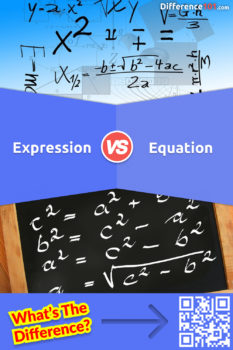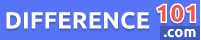# Expression vs. Equation: What is the difference between Expression and Equation?

The difference between expression and equation is often overlooked. Find out how they are different, and how you can use them to your advantage. Read more here.Almost everyone has heard about these two-term, Expression and Equation, in real life, most often in math class. Both terms are used in real-world problems. Both contain numbers and variables. The one difference between both terms is that Expression does not have an equal sign while the Equation always has an equal sign.

Let us take a closer look at Expression vs. Equation:

## What is an Expression?

An expression is a mathematical term with numbers and variables but no equal sign. It is a combination of variables, numbers, and functions. Expression is just like phrases; a phrase is an incomplete sentence and expresses a single thought in language.

👉  Amylose vs. Amylopectin: What is the Difference Between Amylose and Amylopectin?

## What is an Equation?

An equation is a mixture of two expressions, which are joined with each other with the help of an equal sign. Equations form a complete understanding of sentences when written in words.

## Equation vs. Expression similarities

• Whether it is Expression or Equation, both have numbers, variables, and functions
• Both have similarities in learning some common concepts of mathematics
• Both expression and equations are used in computer programming for different tasks.
• Expression of equation calculators have almost the same features and help in solving major algebra problems.
👉  Make vs. Model: What is the difference between Make and Model?

## Pros and Cons of Expression

### Pros of Expression:

• Expressions are used to simplify many problems in mathematics
• Expressions are found in computer programming for different tasks like tasks of inference
• It is used to identify different angles and values in geometry and trigonometry
• It is also used in economics to perform different tasks like finding the cost

### Cons of Expression:

• The expression can only be used to simplify the problem. It cannot solve the problem
•  In writing, expression is an incomplete sentence like phrase without proper meaning

## Pros and cons of Equation

### Pros of Equation:

• The equation is the fastest and simplest way to solve numerical problems
• The equation is used in making silicon chips, compact disks, MRI, and many other things needed by the world
• The equation is also used in aircraft, space programs, and medicine; that is why it is important in our life
• Today’s machines like dryers, cars, washers, and chips are based on mathematical equations and algorithms
👉  Brushed Nickel vs. Satin Nickel: What’s The Difference Between Brushed Nickel And Satin Nickel?

### Cons of Equation:

• An equation can easily solve the problem with smaller numbers, but it is harder to solve with bigger numbers
• Equation with multiple variables is not able to provide a simple numerical value
• Equation which is solved through quadratic formula can result in two values

## Expression vs. Equation vs. Formula

An equation is used to describe the equality between two values. Expression is used to simplify many difficult puzzles of math. While in the formula, the values do not have to be equal, it just describes the relationship between variables.

## Equation vs. Expression FAQs

### What is the difference between an expression and an equation?

An expression is a mathematical phrase that doesn’t have an equal sign between variables or numbers, while equation combines two expressions with an equal sign.

### What is a rational Expression?

Rational expression is defined as the ratio of two polynomials; one is the nominator, other is the denominator.

### What is a linear equation example?

In a linear equation, the highest power of the variable is always 1. For example 3X+3Y=6

### What is algebraic Expression and Equation?

Algebraic expression consists of numbers, variables, and operation symbols. While algebraic equation is a combination of two expressions combined with an equal sign.

### What is an expression in a math equation?

An expression is a number, a variable, an operational symbol, or a combination of both number and variable.

### What are grouping symbols in Expression or Equation, and what do they mean?

The grouping symbols are brackets, braces, and parentheses present in expression or equation. All three of them are used for the same purpose, to provide attention to the number or variable present inside them.

### What is the difference between an expression, an equation, and an identity?

An expression has numbers, variables, and operators, while the equation has two expressions with equal signs in between them. And the identity is the special case of equation in which both expressions are equal in value.

### What is a variable in an equation expression?

A Variable is a symbol that is used to replace or represent a number. For example, in expression 7X+16, X is a variable. And in equation X+7=9, X is variable.

## Conclusion

No matter if it is math expression vs. an equation or algebraic expression vs. equation, both have numbers and variables. The main difference between them is the symbol of the equal sign. expression vs. the equation in math is also differentiated with the help of equal sign. But these both terms play an important role in solving and simplifying some major mathematics problems and also help in inventing some major inventions.Alex Stantor

Alex Stantor is a Sorbonne University (Paris, France) graduate in Philosophy and Data Analysis. Currently, he is an Author and Researcher at Difference 101, he writes articles/blog posts on topics such as "thinking differently" and "the importance of difference". Alex is a passionate advocate of diversity in the workplace and in companies, and diversity and inclusion in corporate communications. He currently lives in Brooklyn.

We will be happy to hear your thoughts